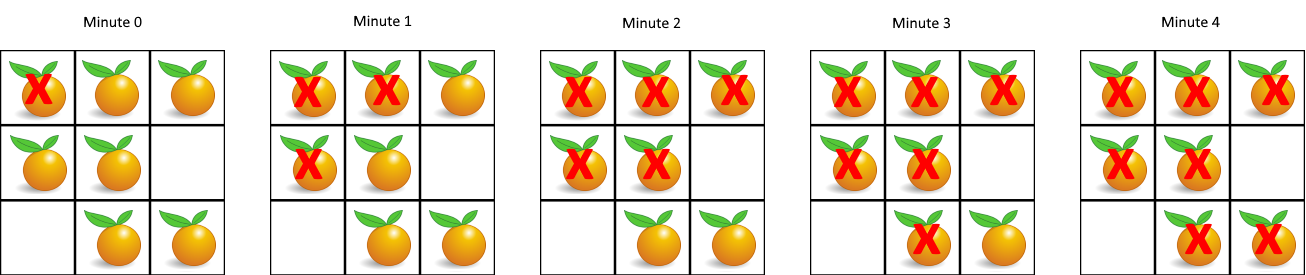# GeetCode Hub

You are given an `m x n` `grid` where each cell can have one of three values:

• `0` representing an empty cell,
• `1` representing a fresh orange, or
• `2` representing a rotten orange.

Every minute, any fresh orange that is 4-directionally adjacent to a rotten orange becomes rotten.

Return the minimum number of minutes that must elapse until no cell has a fresh orange. If this is impossible, return `-1`.

Example 1:```Input: grid = [[2,1,1],[1,1,0],[0,1,1]]
Output: 4
```

Example 2:

```Input: grid = [[2,1,1],[0,1,1],[1,0,1]]
Output: -1
Explanation: The orange in the bottom left corner (row 2, column 0) is never rotten, because rotting only happens 4-directionally.
```

Example 3:

```Input: grid = [[0,2]]
Output: 0
Explanation: Since there are already no fresh oranges at minute 0, the answer is just 0.
```

Constraints:

• `m == grid.length`
• `n == grid[i].length`
• `1 <= m, n <= 10`
• `grid[i][j]` is `0`, `1`, or `2`.

class Solution { public int orangesRotting(int[][] grid) { } }